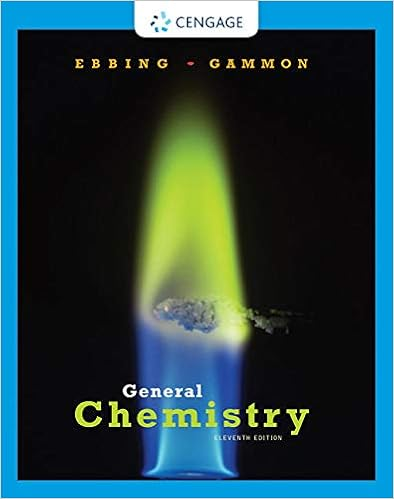# Hydrogen gas exerts a pressure of 466 torr in a

• Test Prep
• 19
• 88% (8) 7 out of 8 people found this document helpful

This preview shows page 2 - 5 out of 19 pages.

##### We have textbook solutions for you!
The document you are viewing contains questions related to this textbook.The document you are viewing contains questions related to this textbook.
Chapter 5 / Exercise 5.55
General Chemistry
Ebbing/GammonExpert Verified
8. Hydrogen gas exerts a pressure of 466 torr in a container. What is this pressure in atmospheres? A) 0.217 atm B) 0.466 atm C) 0.613 atm D) 1.63 atm E) Ans:
4.60 atm
C Difficulty: E 9. The pressure of hydrogen sulfide gas in a container is 35,650 Pa. What is this pressure in torr?
C Difficulty: E 10. The pressure of sulfur dioxide in a container is 159 kPa. What is this pressure in atmospheres?
15900 atm
C Difficulty: E 11. The air pressure in a volleyball is 75 psi. What is this pressure in torr?
C Difficulty: E 12. “The volume of an ideal gas is directly proportional to the number of moles of the gas at constant temperature and pressure” is a statement of _____________ Law. A) Charles's B) Boyle's C) Amontons's D) Avogadro's E) Ans:
Dalton's
D Difficulty: E 13. “The pressure of an ideal gas is inversely proportional to its volume at constant temperature and number of moles” is a statement of __________________ Law.
B Difficulty: E
##### We have textbook solutions for you!
The document you are viewing contains questions related to this textbook.The document you are viewing contains questions related to this textbook.
Chapter 5 / Exercise 5.55
General Chemistry
Ebbing/GammonExpert Verified
Chapter 5: Gases and the Kinetic-Molecular Theory Page 68 14. “The pressure of an ideal gas is directly proportional to its absolute temperature at constant volume and number of moles” is a statement of ________________ Law.
C Difficulty: E 15. “The volume of an ideal gas is directly proportional to its absolute temperature at constant pressure and number of moles” is a statement of ________________ Law.
Dalton's
A Difficulty: E 16. “The total pressure in a mixture of unreacting gases is equal to the sum of the partial pressures of the individual gases” is a statement of __________________ Law. A) Charles's B) Graham's C) Boyle's D) Avogadro's E) Ans:
Dalton's
E Difficulty: E 17. “The rate of effusion of a gas is inversely proportional to the square root of its molar mass” is a statement of ______________________ Law.
Boyle's
B Difficulty: E 18. Which of the lines on the figure below is the best representation of the relationship between the volume of a gas and its pressure, other factors remaining constant?
M
Chapter 5: Gases and the Kinetic-Molecular Theory Page 69 19. Which of the lines on the figure below is the best representation of the relationship between the volume of a gas and its absolute temperature, other factors remaining constant?
Difficulty: M 20. Which of the lines on the figure below is the best representation of the relationship between the volume of a gas and its Celsius temperature, other factors remaining constant? 00bcdeTemperature (°C)aAns: b
Difficulty: M 21. Which of the lines on the figure below is the best representation of the relationship between the volume and the number of moles of a gas, measured at constant temperature and pressure?
•••